# 前言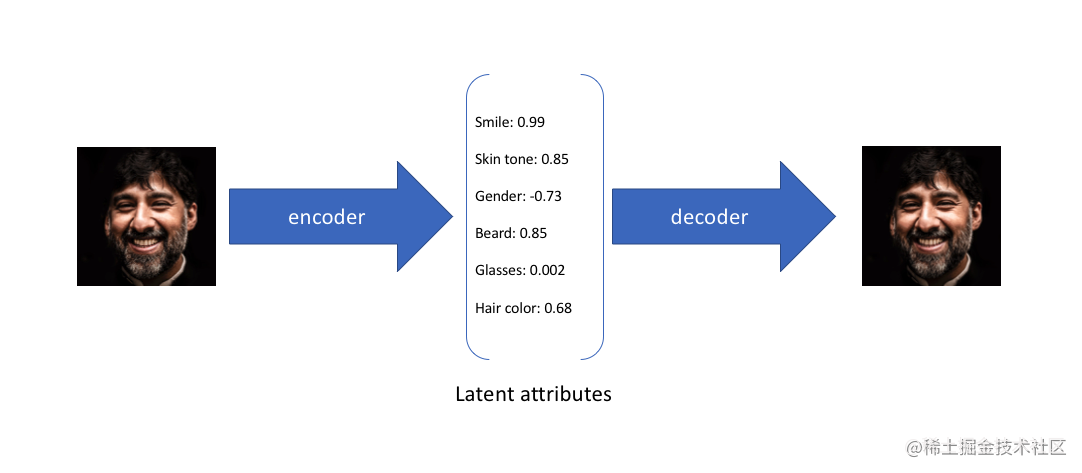(以下相关图来自于jeremy)

# 什么是自编码器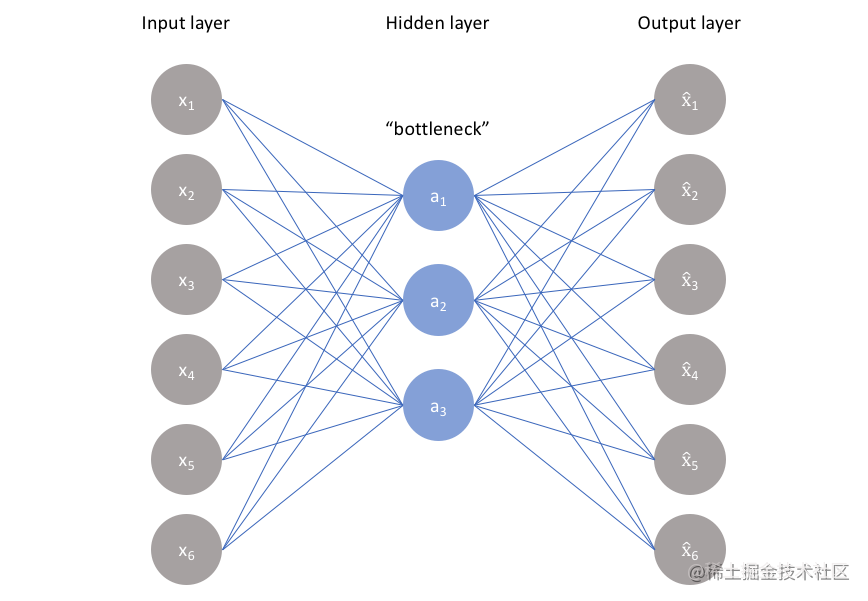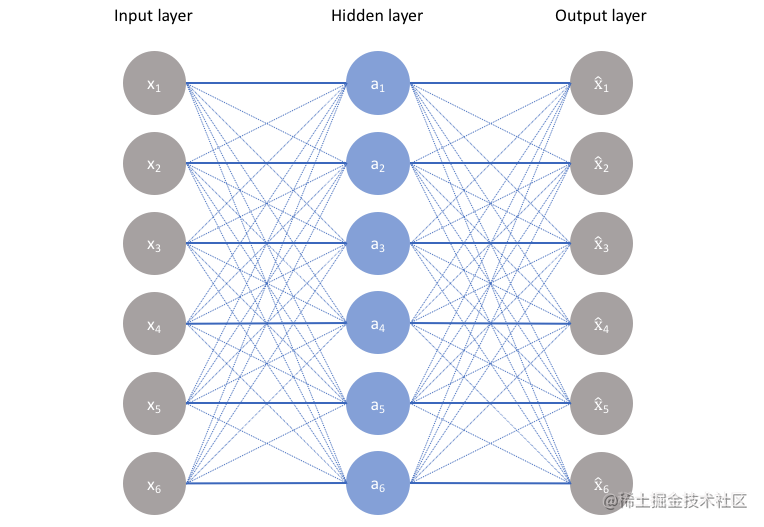## 欠完备自编码器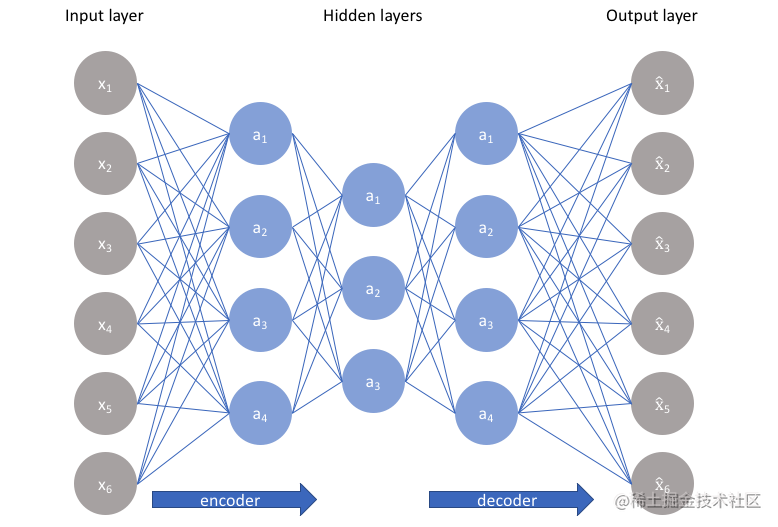$L(x,g(f(x)))$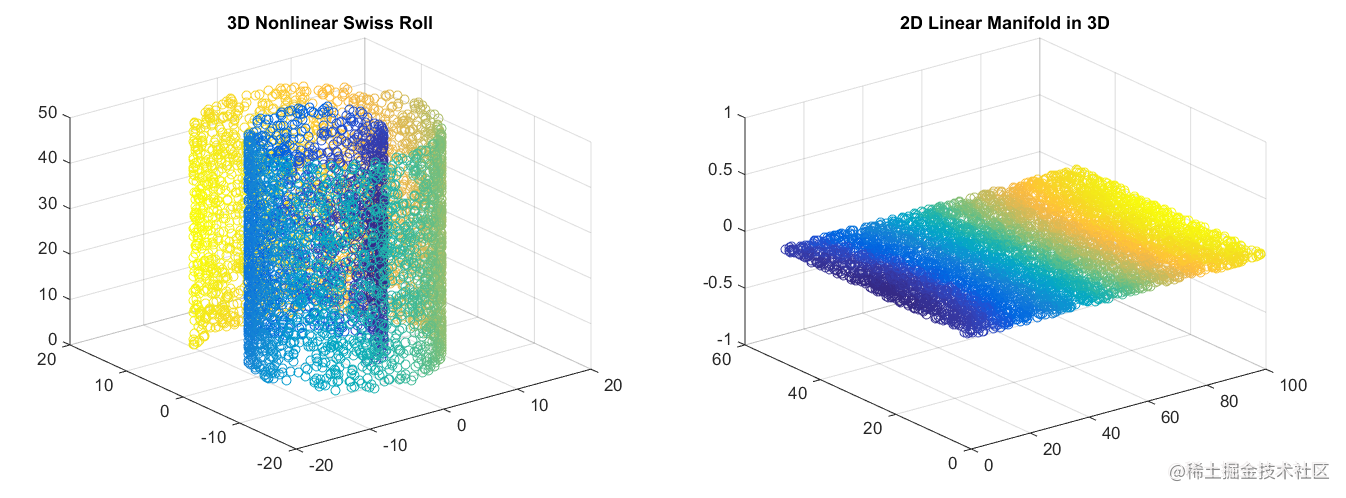Image credit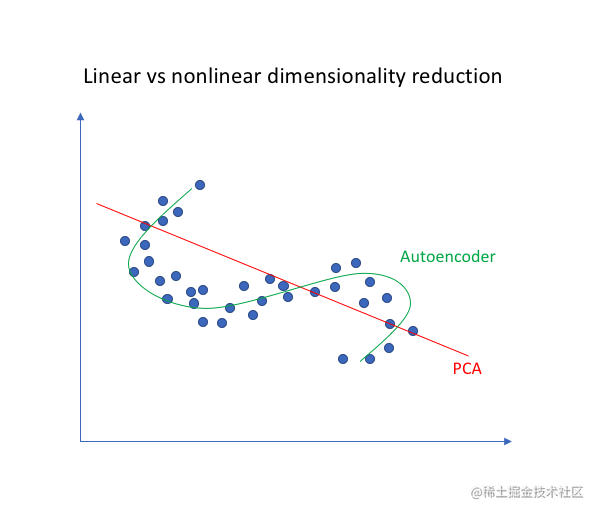class AutoEncoder(nn.Module):
def __init__(self):
super(AutoEncoder, self).__init__()
# 自编码器的编码器构造
self.encoder = nn.Sequential(
nn.Linear(28*28, 128),              # 784 => 128
nn.LeakyReLU(0.1, inplace=True),    # 激活层
nn.Linear(128, 64),                 # 128 => 64
nn.LeakyReLU(0.1, inplace=True),
nn.Linear(64, 12),                  # 64 => 12
nn.LeakyReLU(0.1, inplace=True),
nn.Linear(12, 3),                   # 最后我们得到3维的特征，之后我们在3维坐标中进行展示
)
# 自编码器的解码器构造
self.decoder = nn.Sequential(
nn.Linear(3, 12),                   # 3 => 12
nn.LeakyReLU(0.1, inplace=True),
nn.Linear(12, 64),                  # 12 => 64
nn.LeakyReLU(0.1, inplace=True),
nn.Linear(64, 128),                 # 64 -> 128
nn.LeakyReLU(0.1, inplace=True),
nn.Linear(128, 28*28),              # 128 => 784
nn.Sigmoid(),                       # 压缩值的范围到0-1便于显示
)

def forward(self, x):
hidden = self.encoder(x)                # 编码操作，得到hidden隐含特征
output = self.decoder(hidden)           # 解码操作，通过隐含特征还原我们的原始图
return hidden, output

optimizer = torch.optim.Adam(autoencoder.parameters(), lr=configure['lr'])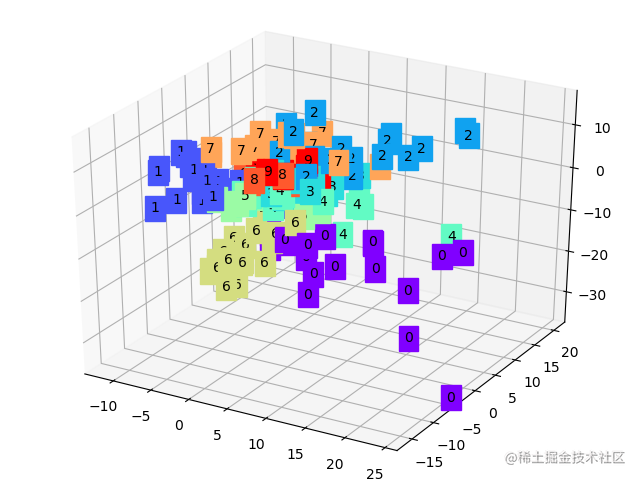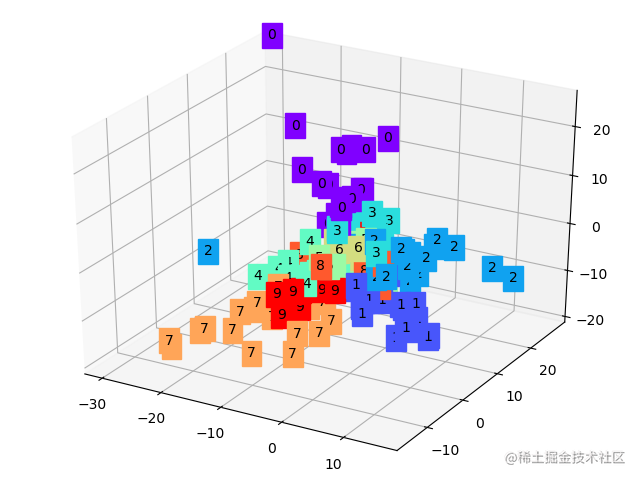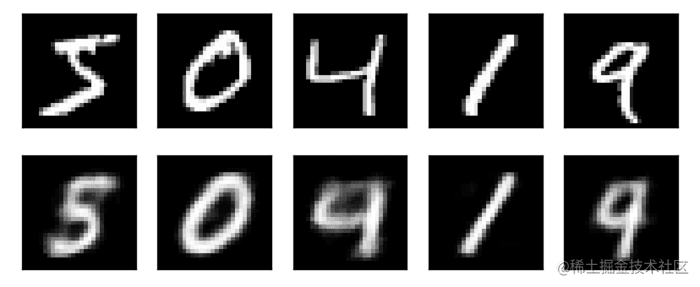## 稀疏自编码器

$L(x,\hat x)+ \lambda\sum\limits_i|a_i^{(h)}|$

$L(x,\hat x)+\sum\limits_{j}KL(\rho||\hat \rho_j)$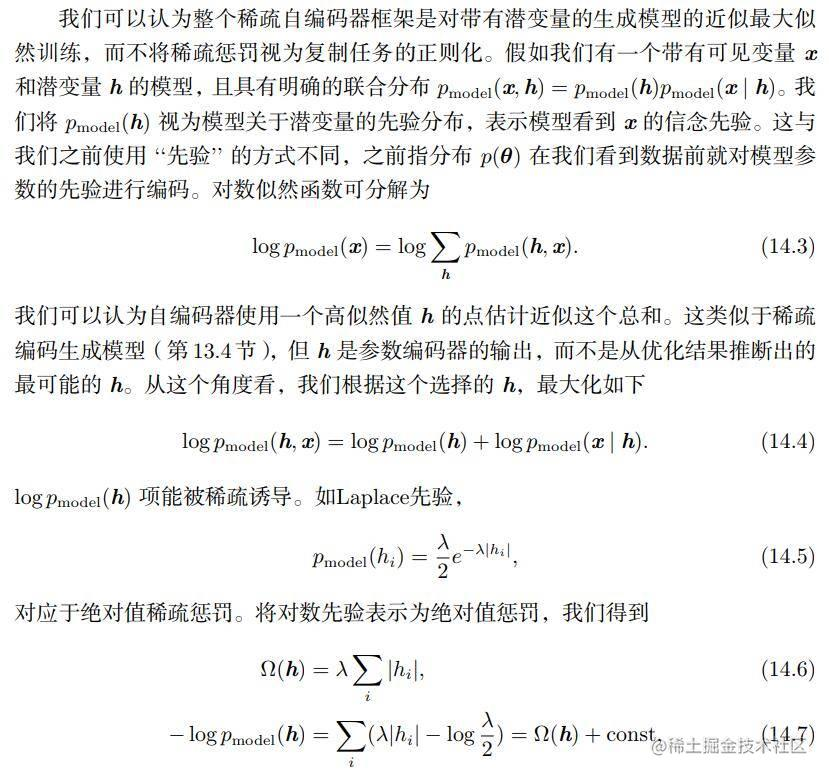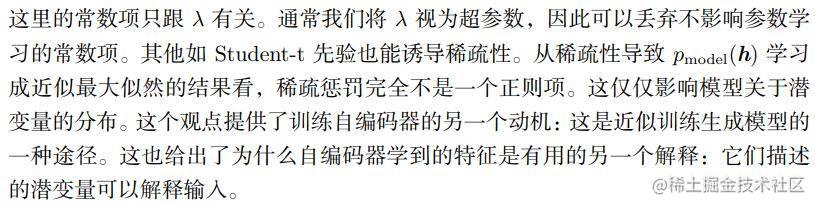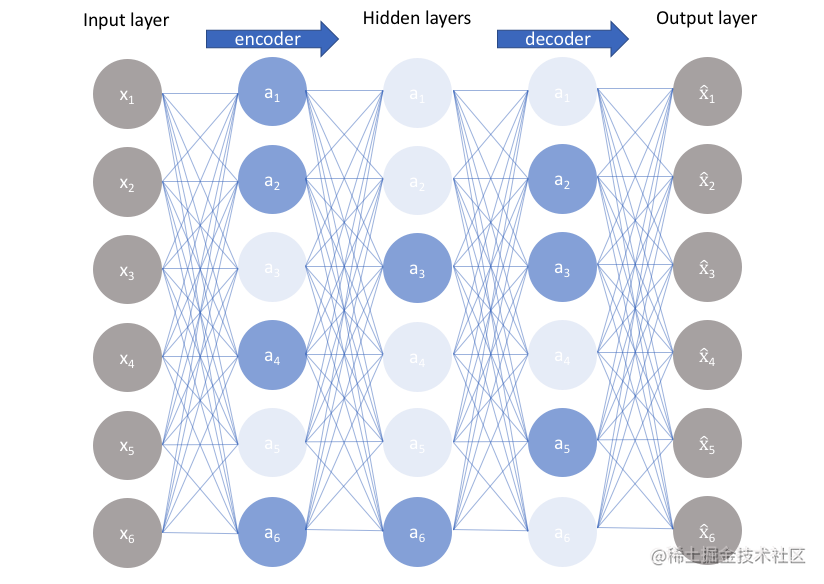# weight_decay为权重衰减系数,我们这里设置为1e-4
# 但这里的惩罚函数为L2

class AutoEncoder(nn.Module):
def __init__(self):
super(AutoEncoder, self).__init__()

self.encoder = nn.Sequential(
nn.Linear(28*28, 28*28),        # 现在所有隐含层的维数是一样的，没有缩小也没有放大
nn.LeakyReLU(0.1, inplace=True),
nn.Linear(28*28, 28*28),
nn.LeakyReLU(0.1, inplace=True),
nn.Linear(28*28, 28*28),
)
self.decoder = nn.Sequential(
nn.Linear(28*28, 28*28),
nn.LeakyReLU(0.1, inplace=True),
nn.Linear(28*28, 28*28),
nn.LeakyReLU(0.1, inplace=True),
nn.Linear(28*28, 28*28),
nn.Sigmoid(),
)

def forward(self, x):
hidden = self.encoder(x)
output = self.decoder(hidden)
return hidden, output

# 撩我吧

• 如果你与我志同道合于此，老潘很愿意与你交流；
• 如果你喜欢老潘的内容，欢迎关注和支持。
• 如果你喜欢我的文章，希望点赞👍 收藏 📁 评论 💬 三连一下~